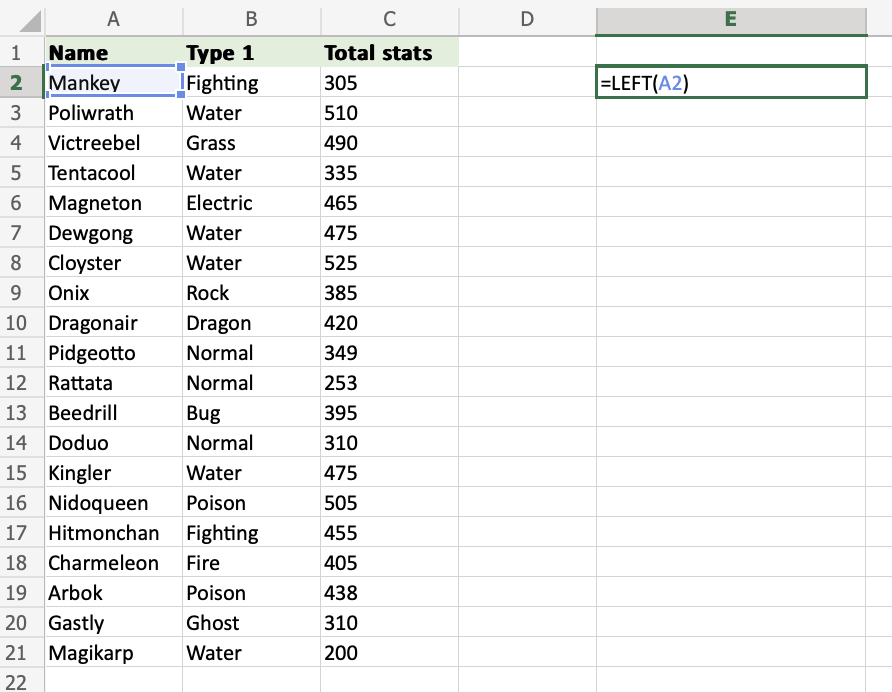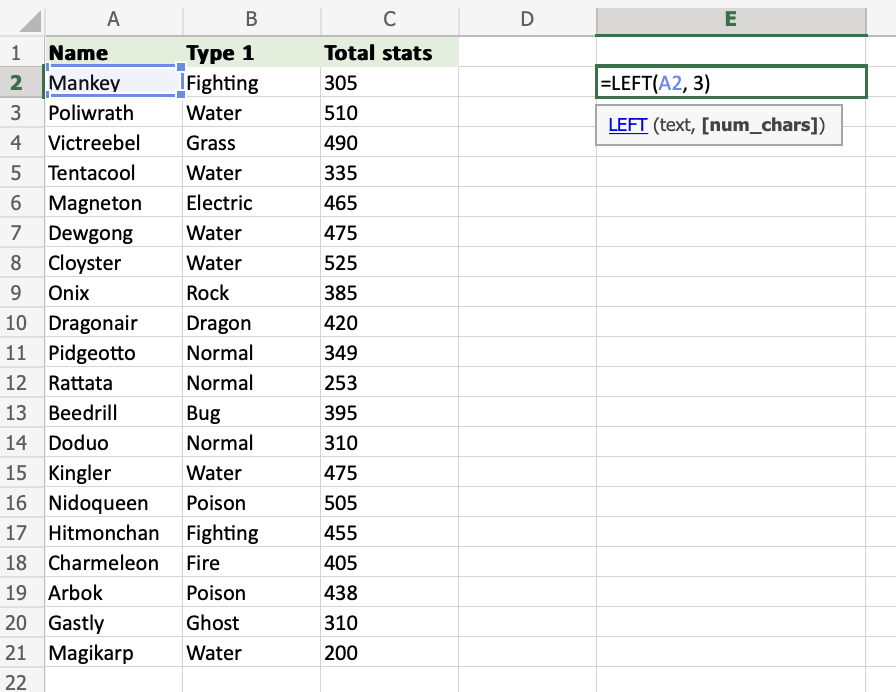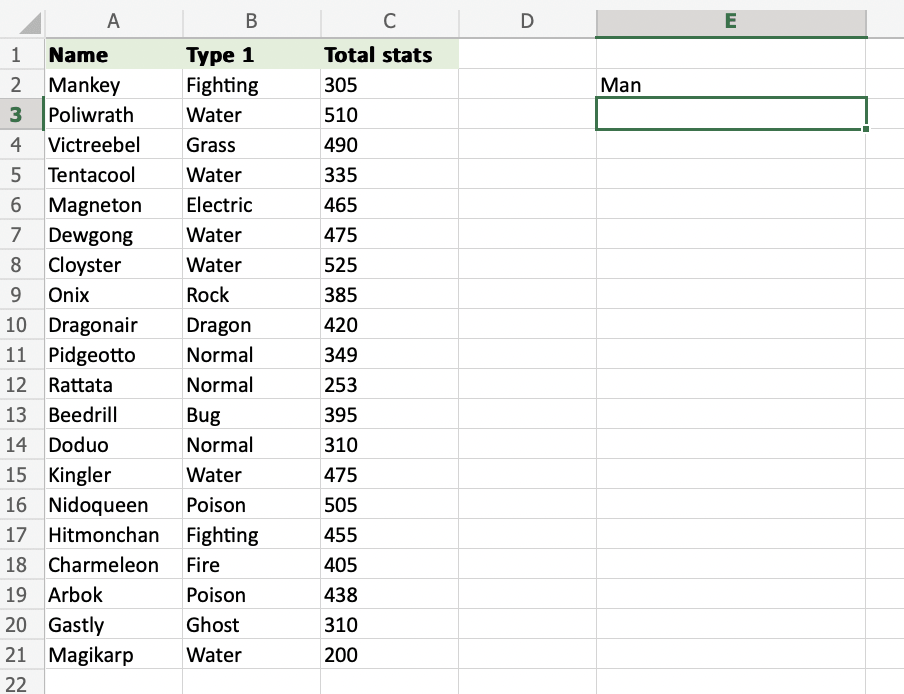# Excel LEFT Function

## LEFT Function

The LEFT function is used to retrieve a chosen amount of characters, counting from the left side of an Excel cell. The chosen number has to be greater than 0 and is set to 1 by default.

It is typed `=LEFT`

If you want to use the function on a single cell, write:

=LEFT(cell)

If you want to use the function on a range of cells, write:

=LEFT(start cell:end cell)

## How to Use LEFT Function

To retrieve values from the left side of an Excel cell, use LEFT.

##### Step 1) Start the LEFT function
1. Select a cell `E2`
2. Type `=LEFT`
3. Double click the LEFT commandCopy Values
##### Follow along the tutorial by trying it yourself!

Copy the values in the example above and try it on your own!

##### Step 2) Enter values to the LEFT function
1. Select a cell (`A2`)
2. Hit enter`=LEFT(A2)` uses the default funcion of LEFT and retrieves the first symbol from the `A2` cell.### How to Use the LEFT Function with a Defined Length of Characters

Learn to use `=LEFT` to get more than one character.

##### Step 1) Start the LEFT function
1. Select a cell `E2`
2. Type `=LEFT`
3. Double click the LEFT command

Note: The different parts of the function are separated by a symbol, like comma `,` or semicolon `;`

The symbol depends on your Language Settings.##### Step 2) Select cells and set the number of characters
1. Enter the cell name (`A2`) and define the length of characters you want to retrieve, using a `,` as a delimiter (`A2,3`)
2. Hit enter

The function returns the first 3 characters from cell `A2`.### How to use the LEFT Function on a Range of Cells

##### Step 1) Start the LEFT function
1. Select a cell `E2`
2. Type `=LEFT`
3. Double click the LEFT command##### Step 2) Select cells and number of characters
1. Select the cells (`A2:A4`) and define the length of characters you want to retrieve, using a `,` as a delimiter (`A2:A4,3`)
2. Hit enter

The function returns the first 3 characters from each cell within the range `A2:A4`.